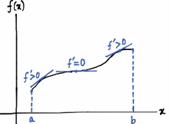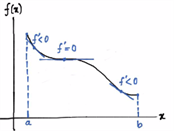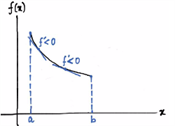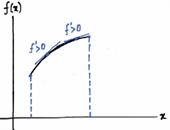# Increasing And Decreasing Functions & Monotonicity

Increasing and Decreasing Functions

Before explaining the increasing and decreasing function along with monotonicity, let us understand what functions are. A function is basically a relation between input and output such that, each input is related to exactly one output.

Functions can increase, decrease or can remain constant for intervals throughout their entire domain. Functions are continuous and differentiable in the given intervals.

An interval in Maths is defined as a continuous/connected portion on the real line. Since it is a “portion of a line”, it basically is a line segment which has two endpoints. So, an interval has two endpoints. Easy to keep track, let’s name our interval and the endpoints and in an interval, assume any two points viz.

$$\begin{array}{l} x_1 ~and ~x_2 \end{array}$$
such that
$$\begin{array}{l} x_1 < x_2 \end{array}$$
. Now, there can be a total of four different cases:
If
$$\begin{array}{l} f(x_1) \leq f(x_2)\end{array}$$
, the function is said to be non-decreasing in l
If
$$\begin{array}{l} f(x_1) \geq f(x_2)\end{array}$$
, the function is said to be non-increasing in l
If
$$\begin{array}{l} f(x_1) > f(x_2)\end{array}$$
, the function is said to be decreasing (strictly) in l
If
$$\begin{array}{l} f(x_1) < f(x_2)\end{array}$$
, the function is said to be increasing (strictly) in l

This increasing or decreasing behaviour of functions is commonly referred to as monotonicity of the function. A monotonic function is defined as any function which follows one of the four cases mentioned above. Monotonic basically has two terms in it. Mono means one and tonic means tone. Together, it means, “in one tone”. When we tell that a function is non-decreasing, does it mean that it is increasing? No. It can also mean the function not varying at all! In other words, function having a constant value for some interval. Never confuse non-decreasing with increasing. That was the definition of increasing and decreasing functions. Let us now see how to know where and in which way the function is behaving.

## Test for increasing and decreasing functions

Let us now use derivative of a function to determine the behaviour of a function. To test the monotonicity of a function

$$\begin{array}{l} f \end{array}$$
, we first calculate it’s derivative
$$\begin{array}{l} f’ \end{array}$$
. There is a small catch here. Before starting the test, make sure that is continuous in the interval
$$\begin{array}{l} [a, b] \end{array}$$
and differentiable in
$$\begin{array}{l} (a, b)\end{array}$$
. So, for all of the four cases discussed in previous heading, we have tests as:
For function to be non-decreasing in
$$\begin{array}{l} l, f'(x) \geq 0, \forall ~x \in (a, b) \end{array}$$

For function to be non-increasing in
$$\begin{array}{l} l, f'(x) \leq 0, \forall ~x \in (a, b) \end{array}$$

For function to be decreasing/strictly decreasing in
$$\begin{array}{l} l, f'(x) < 0, \forall ~x \in (a, b) \end{array}$$

For function to be increasing/strictly increasing in
$$\begin{array}{l} l, f'(x) > 0, \forall ~x \in (a, b)\end{array}$$
<

Let us see examples of each case.

 Nature of graph of f Cases Behaviour Testf(x1 ) ≤f(x2) Non – decreasing f'(x) ≥ 0,  ∀ x ∈ (a, b)f(x1) ≥ f(x2) Non – increasing f'(x)  ≤  0,  ∀ x ∈ (a, b)f(x1) > f(x2) Decreasing/ Strictly decreasing f'(x) < 0,  ∀ x ∈ (a, b)f(x1) < f(x2) Increasing/ Strictly Increasing f'(x) > 0,  ∀ x ∈ (a, b)

That was increasing and decreasing functions, monotonic functions and monotonicity explained. This concept is particularly very useful for drawing graphs of functions. To learn more, visit www.byjus.com and find new content every day. Download BYJU’S-The Learning App from Google Play Store to explore more!